# Calibration with a square wave (step) signal

Güralp digitisers provide calibration signal generators to help you set up your sensors. Calibration is most easily done through a PC running Güralp's Scream! software, but you can also do it using a hand-held control unit (HCU) and an oscilloscope.

Depending on the digitiser type, sine-wave, step and broadband noise signal generators may be available. In this article, we will use a step input signal to determine sensor characteristics for a conventional-response velocity instrument. Step calibration is less useful for hybrid-response instruments.

1. In Scream!’s main window, right-click on the digitiser’s icon and select Control…. Open the Calibration pane.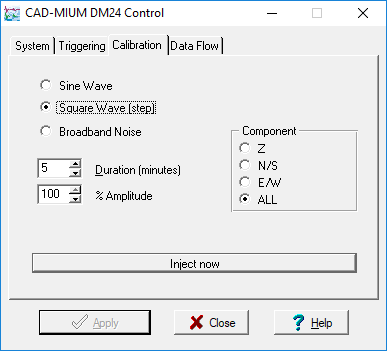2. Select the calibration channel corresponding to the instrument, and choose Square Wave (step). Select a suitable amplitude, make any other choices you require, and click Inject now. A new data stream, ending Cn (where n = 0 – 7) or MB, should appear in Scream!’s main window. This stream contains the returned calibration signal.
3. Open a WaveView window on the calibration signal and the returned streams by selecting them and double-clicking. The streams should display the calibration signal combined with the sensors’ own measurements. If you cannot see the calibration signal, zoom into the WaveView using the scaling icons at the top left of the window or the cursor keys.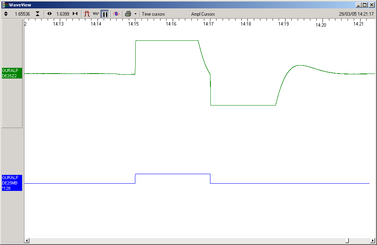4. If the returning signal is saturated (i.e. the top and bottom of the waveform is flat, as in the picture above), retry using a calibration signal with lower amplitude, until the entire curve is visible in the WaveView window.
5. If you need to scale one, but not another, of the traces, right-click on the trace and select Scale…. You can then type in a suitable scale factor for that trace.
6. Click on Time Cursors in the top right hand corner of the window. A white square will appear inside the WaveView at the top left. This is, in fact, two superimposed cursors.
7. Drag one cursor across to the beginning of the returning signal.
8. Drag the other across to be level with the first peak.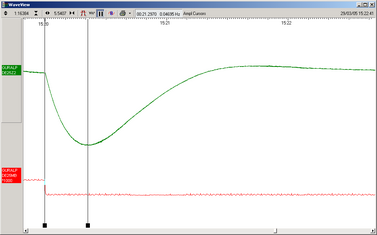9. The Time Cursors button will now be displaying a value, which is the time period indicated in seconds.
10. The rise time to the first peak, Tpk, is related to the long-period corner frequency Tp by the equation:
Tpk = Tp / 5.656
The example above was created using a 120 s sensor, Tpk = 120 / 5.656 = 21.216 s.
The measured value, 21.297 s, agrees with the theoretical result to within the margin of error of the experiment.
11. Click on Time Cursors once more, to remove them, and click on Ampl Cursors in the top right hand corner of the window.
12. Drag one cursor down to be level with the zero point of the returning signal trace.
13. Drag the other down to be level with the first peak.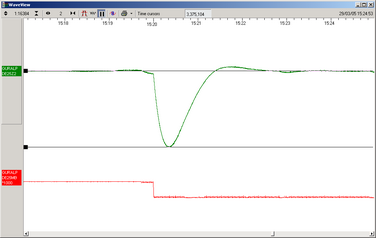14. The Ampl Cursors button will now be displaying a value, which is the strength of the returning signal in counts. If you have used the Scale… option, you will need to take the scale factor into account to produce the correct number of counts.
15. Convert to volts using the µV/Bit value given on the digitiser’s calibration sheet for the input sensitivity.
16. Measure the calibration input signal in the same way.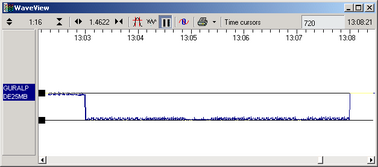17. Convert to volts using the µV/Bit value given on the digitiser’s calibration sheet for the calibration channel.
18. Convert this voltage to a calibration acceleration using the equation
a = V / RK
where R is the value of the calibration resistor, and K is the feedback coil constant.
19. The calibration acceleration is related to sensor parameters by the equation:
V0 = 0.3244H Vcal / Rcal ωn K
where V0 is the voltage of the first peak and H is the sensitivity of the component in V/ms-1. For a velocity system (ζ = 0.707), ωn = 2πb, where b is the imaginary part of the complex conjugate pair of the long period corner. The equation can therefore be rewritten as:
V0 = aH / 19.369b
where a is the calibration acceleration, above. H is given on the main calibration sheet. To find b, look for the single complex conjugate pole in the Poles and Zeros table. For example, the Poles column for a 120 s 3T might look like
```POLES (HZ)
-5.89 x 10-3 ± 	5.89 x 10-3j
-180
-180
-80 ```

In this case, the value of b is 5.89 × 10-3. If there are more than one complex conjugate pair, the one with the smallest absolute value should be used. To compute the absolute value, add the squares of the real and imaginary parts and take the square root of the result. (It is not necessary to take the root when merely comparing.)

20. To obtain the velocity responsivity, the equation above can be rearranged to
H = 19.369bV0 / a
The measured sensitivity for a 1 Hz sinusoidal calibration signal is given on the sensor’s calibration sheet. Since the instrument has a flat velocity response over the pass-band, this method should give the same result.

You can view our case studies to find out more about how our instrumentation is used around the world.

### Address

Guralp Systems Limited
Midas House
Calleva Park
Aldermaston
Reading
RG7 8EA, UK

Tel: +44 118 981 9056
Fax: +44 118 981 9943
E-Mail: sales@guralp.com

### Applications

Seismology

Civil

Energy

Other Applications

JoomShaper